Updated On : Dec-13,2019 Time Investment : ~10 mins

Matplotlib recognizes the following formats to specify a color:

• an RGB or RGBA tuple of float values in [0, 1] (e.g., (0.1, 0.2, 0.5) or (0.1, 0.2, 0.5, 0.3)). RGBA is short for Red, Green, Blue, Alpha;
• a hex RGB or RGBA string (e.g., '#0F0F0F' or '#0F0F0F0F');
• a string representation of a float value in [0, 1] inclusive for gray level (e.g., '0.5'); one of {'b', 'g', 'r', 'c', 'm', 'y', 'k', 'w'};
• a X11/CSS4 color name;
• a name from the xkcd color survey; prefixed with 'xkcd:' (e.g., 'xkcd:sky blue'); one of {'tab:blue', 'tab:orange', 'tab:green', 'tab:red', 'tab:purple', 'tab:brown', 'tab:pink', 'tab:gray', 'tab:olive', 'tab:cyan'} which are the Tableau Colors from the 'T10' categorical palette (which is the default color cycle);
• a "CN" color spec, i.e. 'C' followed by a single digit, which is an index into the default property cycle (matplotlib.rcParams['axes.prop_cycle']); the indexing occurs at artist creation time and defaults to black if the cycle does not include color. "Red", "Green" and "Blue", are the intensities of those colors, the combination of which span the colorspace.

How "Alpha" behaves depends on the zorder of the Artist. Higher zorder Artists are drawn on top of lower Artists, and "Alpha" determines whether the lower artist is covered by the higher. If the old RGB of a pixel is RGBold and the RGB of the pixel of the Artist being added is RGBnew with Alpha alpha, then the RGB of the pixel is updated to RGB = RGBOld (1 - Alpha) + RGBnew Alpha. Alpha of 1 means the old color is completely covered by the new Artist, Alpha of 0 means that pixel of the Artist is transparent.

```import numpy as np
import matplotlib.pyplot as plt
import matplotlib as mpl

th = np.linspace(0, 2*np.pi, 128)

def demo(sty):
mpl.style.use(sty)
fig, ax = plt.subplots(figsize=(3, 3))

ax.set_title('style: {!r}'.format(sty), color='C0')

ax.plot(th, np.cos(th), 'C1', label='C1')
ax.plot(th, np.sin(th), 'C2', label='C2')
ax.legend()

demo('default')
demo('seaborn')
plt.show()
```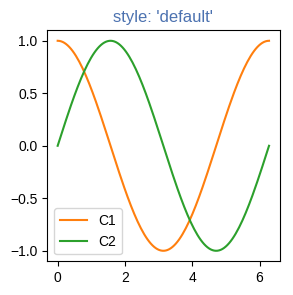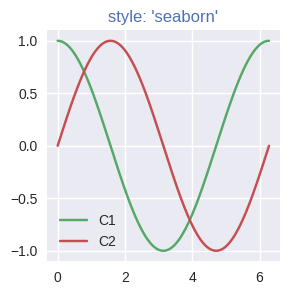```import matplotlib._color_data as mcd
import matplotlib.patches as mpatch

overlap = {name for name in mcd.CSS4_COLORS
if "xkcd:" + name in mcd.XKCD_COLORS}

fig = plt.figure(figsize=[4.8, 16])
ax = fig.add_axes([0, 0, 1, 1])

for j, n in enumerate(sorted(overlap, reverse=True)):
weight = None
cn = mcd.CSS4_COLORS[n]
xkcd = mcd.XKCD_COLORS["xkcd:" + n].upper()
if cn == xkcd:
weight = 'bold'

r1 = mpatch.Rectangle((0, j), 1, 1, color=cn)
r2 = mpatch.Rectangle((1, j), 1, 1, color=xkcd)
txt = ax.text(2, j+.5, '  ' + n, va='center', fontsize=10,
weight=weight)
ax.axhline(j, color='k')

ax.text(.5, j + 1.5, 'X11', ha='center', va='center')
ax.text(1.5, j + 1.5, 'xkcd', ha='center', va='center')
ax.set_xlim(0, 3)
ax.set_ylim(0, j + 2)
ax.axis('off')
plt.show()
```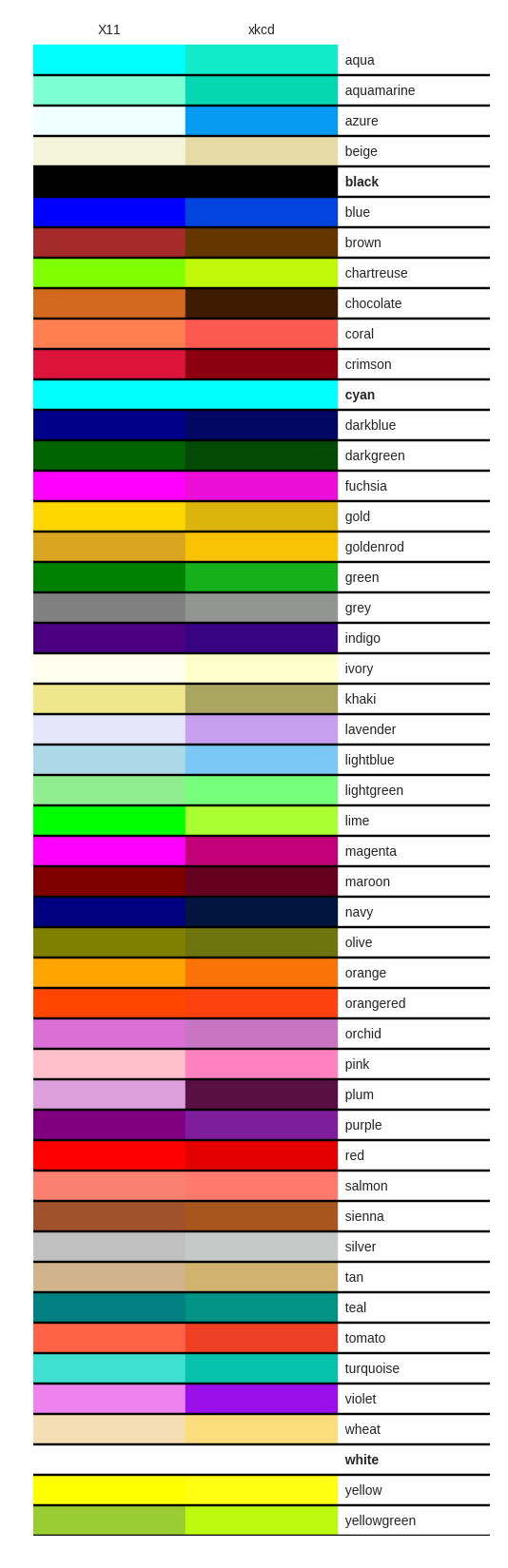```import matplotlib.pyplot as plt
import numpy as np

t = np.linspace(0.0, 2.0, 201)
s = np.sin(2 * np.pi * t)

# 1) RGB tuple:
fig, ax = plt.subplots(facecolor=(.18, .31, .31))
# 2) hex string:
ax.set_facecolor('#eafff5')
# 3) gray level string:
ax.set_title('Voltage vs. time chart', color='0.7')
# 4) single letter color string
ax.set_xlabel('time (s)', color='c')
# 5) a named color:
ax.set_ylabel('voltage (mV)', color='peachpuff')
# 6) a named xkcd color:
ax.plot(t, s, 'xkcd:crimson')
# 7) Cn notation:
ax.plot(t, .7*s, color='C4', linestyle='--')
# 8) tab notation:
ax.tick_params(labelcolor='tab:orange')

plt.show()
```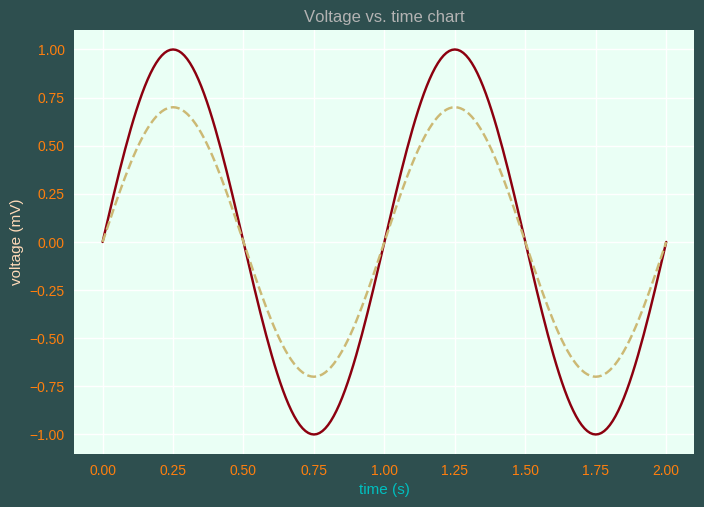```import matplotlib.pyplot as plt
from matplotlib import colors as mcolors

colors = dict(mcolors.BASE_COLORS, **mcolors.CSS4_COLORS)

# Sort colors by hue, saturation, value and name.
by_hsv = sorted((tuple(mcolors.rgb_to_hsv(mcolors.to_rgba(color)[:3])), name)
for name, color in colors.items())
sorted_names = [name for hsv, name in by_hsv]

n = len(sorted_names)
ncols = 4
nrows = n // ncols

fig, ax = plt.subplots(figsize=(9, 8))

# Get height and width
X, Y = fig.get_dpi() * fig.get_size_inches()
h = Y / (nrows + 1)
w = X / ncols

for i, name in enumerate(sorted_names):
row = i % nrows
col = i // nrows
y = Y - (row * h) - h

xi_line = w * (col + 0.05)
xf_line = w * (col + 0.25)
xi_text = w * (col + 0.3)

ax.text(xi_text, y, name, fontsize=(h * 0.5),
horizontalalignment='left',
verticalalignment='center')

ax.hlines(y + h * 0.1, xi_line, xf_line,
color=colors[name], linewidth=(h * 0.6))

ax.set_xlim(0, X)
ax.set_ylim(0, Y)
ax.set_axis_off()

top=1, bottom=0,
hspace=0, wspace=0)
plt.show()
```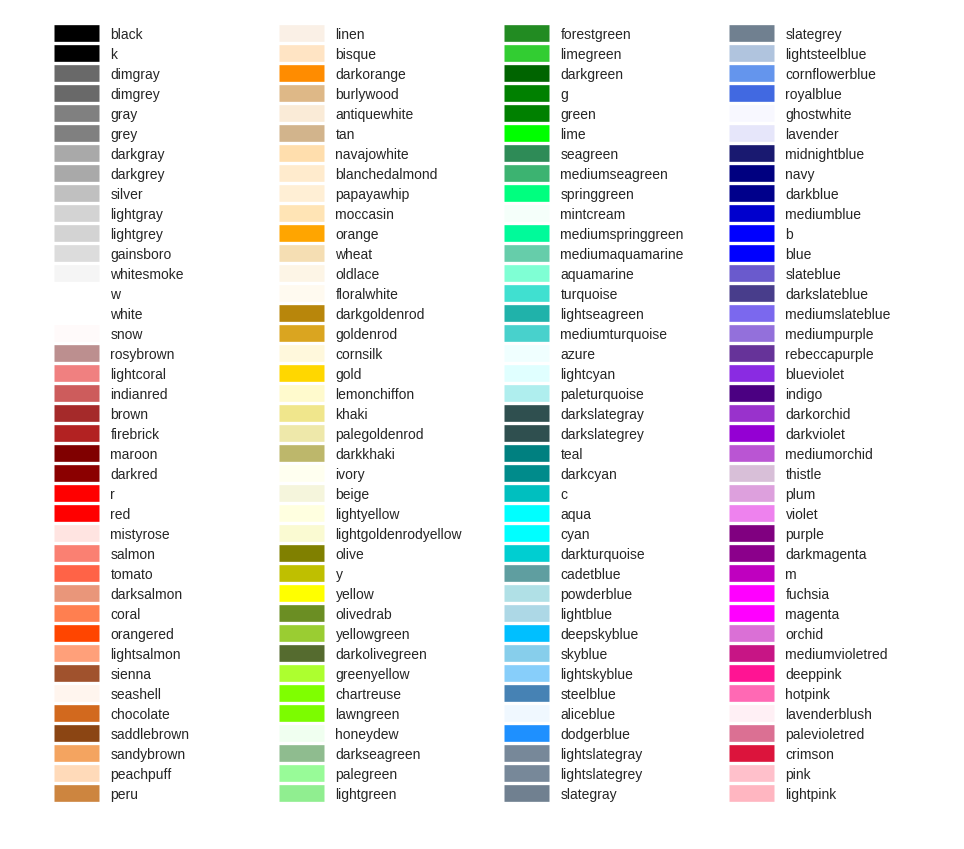Dolly Solanki

## Stuck Somewhere? Need Help with Coding? Have Doubts About the Topic/Code?

When going through coding examples, it's quite common to have doubts and errors.

If you have doubts about some code examples or are stuck somewhere when trying our code, send us an email at coderzcolumn07@gmail.com. We'll help you or point you in the direction where you can find a solution to your problem.

You can even send us a mail if you are trying something new and need guidance regarding coding. We'll try to respond as soon as possible.

## Want to Share Your Views? Have Any Suggestions?

If you want to

• provide some suggestions on topic
• share your views
• include some details in tutorial
• suggest some new topics on which we should create tutorials/blogs
Please feel free to contact us at coderzcolumn07@gmail.com. We appreciate and value your feedbacks. You can also support us with a small contribution by clicking DONATE.